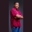Related Tags

scala
rectangle
area
communitycreator

# How to get the area of a rectangle in ScalaTheodore Kelechukwu Onyejiaku

### Overview

The area of a rectangle is the space occupied by the rectangle. It is the product of its length and breadth. In other words, we get the area after multiplying the length of the rectangle with its width. The length of a rectangle refers to the longest side of the rectangle. On the other hand, the breadth, which is also known as the width, is the shortest part.

### Syntax

length *  breadth
Syntax to get the area of a rectangle in Scala

### Parameters

length: This is the length of the rectangle.

breadth: This is the breadth of the rectangle.

### Return value

A float value is returned, which is the product of the length and breadth specified.

### Code example

object Main extends App {
// create some lengths
var length1 = 23
var length2 = 4.5F
var length3 = 50

// print the areas
}
Get the area of a rectangle in Scala

### Explanation

In the code above,

• Lines 3-5: We declare and initialize the lengths of the rectangles.
• Lines 8-10: We declare and initialize the breadths of the rectangles.
• Lines 13-15: We print the products of the lengths and breadths. The results are the areas of the rectangles with such lengths and breadths.

RELATED TAGS

scala
rectangle
area
communitycreator

CONTRIBUTORTheodore Kelechukwu Onyejiaku
RELATED COURSES

View all Courses

Keep Exploring

Learn in-demand tech skills in half the time